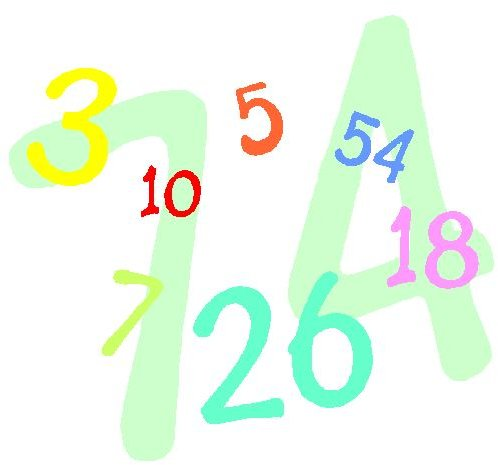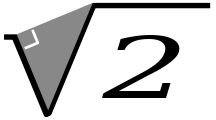# Rational and Irrational Numbers: What’s the Difference, Including a Discussion of Pi

## Rational and Irrational Numbers

There's a math cartoon that makes me laugh in which i, an imaginary number, tells pi, an irrational number, to "be rational!" Pi, of course,tells i to "get real!"

An irrational number is one that cannot be expressed as the ratio of two integers; the existence of such numbers was discovered by one of Pythagoras' students in the 5th century BC (although there have been other claims to the discovery as well). The story goes that Pythagoras was so upset by his student's discovery that, unable to disprove it, he had the student thrown overboard and drowned!

The set of irrational real numbers is uncountably infinite; in layman's terms, there are infinitely more irrational numbers than there are integers.

## Examples of Rational and Irrational Numbers

Rational numbers are easy to find. Any integer (such as 7 or -3) is a rational number. So is any decimal with a finite number of digits, or even a decimal with an infinite number of digits if it is a repeating number (such as 10/3, which we could go on forever writing as 3.3333333333..) Mathematically, we say that the rational numbers include all terminating or repeating decimals.

An irrational number, on the other hand, goes on forever and is not repeating. Commonly used irrational numbers include pi (the ratio between a circle's circumference and its diameter), e (the base of the natural logarithm), and the square root of two.

## Proving a Number is Irrational

Here is a sample proof to show that a number is irrational; its first known appearance is in Euclid's Elements.Suppose that √2 is a rational number; then there exist two integers a and b such that a/b = √2. There are an infinite number of choices for a and b; we choose the two smallest. In other words, we choose a and b that are relatively prime – they have no factors in common; we can also say that a/b is irreducible.

Now, if a/b=√2, then squaring both sides gives us that a2/b2 = 2. Rewriting, we get that a2 = 2b2. Clearly 2b2 is an even number (since it is a multiple of two), which means that a2 (being equal to it) must be even as well; it then follows that a is even because the square of an odd number is another odd number.

If a is even, it can be written as twice another number; call that number k. Then we have a=2k and a2 = 4k2. Substituting into the above equation, we have 4k2 = 2b2; dividing both sides by two gives us b2 = 2k2, so b2, and thus b, are even numbers.

But if a and b are both even, then a/b is not irreducible, which contradicts our original assumption, so no such a and b exist and √2 must be irrational.

This is known as proof by infinite descent, a form of proof by contradiction: we assume that a statement X is true, show that X implies some smallest possible example, and then prove that there must be a smaller example (and, through the same logic, an infinite number of smaller examples); thus, our original assumption must have been incorrect, so X is actually false.

## Random Trivia

Pi, the best known irrational number, has been calculated to trillions of digits. Many people memorize some digits of pi for fun; one mnenomic for doing so is the sentence "How I need a drink, alcoholic of course, after the heavy lectures involving quantum mechanics", in which the number of letters in each word gives you the next digit of pi (3.14159265358979).

According to the Guinness Book of World Records, the record for memorizing pi is held by Chao Lu of China, who recited it to 67,890 decimal places, in 2005. In 2006, Akira Haraguchi recited 83,431 digits, but despite his action being filmed in a public hall, the Guiness people have not yet accepted his feat.

Pi day is celebrated on March 14, generally at 1:59.

## References

Guinness Book of World Records